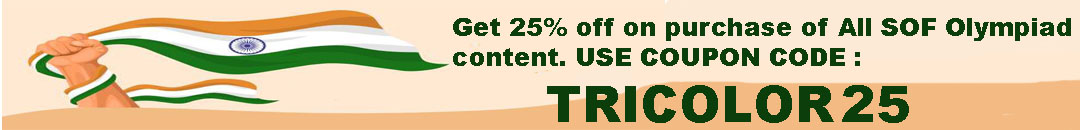# User ForumSubject :IMO    Class : Class 3

Class : Class 1

85+75689+4356-10012 = 70118

Subject :IMO    Class : Class 4

Subject :IMO    Class : Class 2

Subject :IMO    Class : Class 6

## Ans 1:

Class : Class 7
P=l+b×2 L=5w B=w =5w+w×2 =6w×2 =12w cm

## Ans 2:

Class : Class 7
Breadth=w, Length is 5 times more, Therefore, length = 5w Therefore, perimeter=2(5w+w) =2*6w =12w Hence, b is the correct answer

Class : Class 4

Class : Class 6

## Ans 5:

Class : Class 9
6w

Subject :IMO    Class : Class 6

## Ans 1:

Class : Class 7
The answer is d because 2/4 is smaller than 2/3.

Class : Class 1

Class : Class 8
yeah

## Ans 4:

Class : Class 6
yeah....crrct

Subject :IMO    Class : Class 6

## Ans 1:

Class : Class 8
There is a problem in this question. Please correct this.

Class : Class 6
its wrong!!!

## Ans 3:

Class : Class 7
Then the calculation will turn to 20 +8/4x3x2, =20+2x3x2, =20+6x2, =20+12 =32, so there is a problem in the question

Class : Class 7

## Ans 5:

Class : Class 7
The divisions sign is used in 2 places, but I think it means subtraction because '-' meaning subtraction is not exactly coding anything...And in that case 20 8/4-3x2=20 2-6=16...Which is not an options for whatever weird reason

## Ans 6:

Class : Class 4
yes there is an error in the question

Class : Class 7

## Ans 8:

Class : Class 6
How can you change 2 to 3? and how can you mark division symbol as addition and subtraction? SOF DON'T DO SUCH MISTAKES OR WE'LL HAVE TO REPORT IT TO sofworld.org

## Ans 9:

Class : Class 9
yes there is a problem in the question

## Ans 10:

Class : Class 6
answer should be 16 as - coded for subtraction doesn't really code anything so it is 22-6=16

## Ans 11:

Class : Class 6
Ya there is a problem...............answer is 32 you seee

## Ans 12:

Class : Class 6
There is problem in the Q as it mentions division sign represents both addition and substraction

Class : Class 8

## Ans 14:

Class : Class 7
division sign is both addition and subtraction....whyyyyyyy

## Ans 15:

Class : Class 8
There is a damn problem with this question .....Is this what I paid for?!?

Class : Class 7

Class : Class 8

Class : Class 8

Class : Class 8

## Ans 20:

Class : Class 7
Why do things like this ALWAYS happen with sof?

## Ans 21:

Class : Class 8
the answer can be 31 and 13

Class : Class 5

## Ans 23:

Class : Class 6
yes pls correct the problem...

## Ans 24:

Class : Class 9
Yes there is a problem in the question

## Ans 25:

Class : Class 8
actually! it is 32...........plz correct it

## Ans 26:

Class : Class 8
yes, and should be 32, which is not an option. Pl. correct this

## Ans 27:

Class : Class 8
there is problem in the question.....pls correct it

## Ans 28:

Class : Class 6
WHY ARE YOU CONFUSING US AND WHY ARE YOU NOT PROVIDING CORRECT QUESTIONS AND WHY ARE YOU MAKING US LOSE OUR MARKS???????? SOF this is WRONG!!!!!!!!!!!!!!!!!

Class : Class 7

## Ans 30:

Class : Class 8
the division sign represents both addition and subtraction and we can get to different answers

Subject :IMO    Class : Class 10

Class : Class 1

In ΔAED
AE D + ∠EAD + 90° = 180° (Angle sum property)
⇒ ∠AED + ∠EAD = 90°
⇒ ∠EAD = 90° – ∠AED ...(i)
Also, ∠EAD = (50° + ∠AFB) ...(ii) (Exterior angle property)
From (i) and (ii), we have
50° + ∠AFB = 90° – ∠AED
⇒ ∠AFB + ∠AED = 90° – 50° = 40°
⇒ ∠AED + ∠CFD = 40°

## Ans 2:

Class : Class 10
C

Subject :IMO    Class : Class 5

Class : Class 6

Class : Class 6
5

Class : Class 5
D

## Ans 4:

Class : Class 6

Subject :IMO    Class : Class 6

## Ans 1:

Class : Class 8
ss

Class : Class 1

In the 1st column, 2 X 1 + 1 = 3,

In the 2nd column, 12 X 9 + 9 = 117

Similarly, In the 3rd column, 7 X 14 + 7 = 105

Hence, the missing number is 7.

## Ans 3:

Class : Class 9

Subject :IMO    Class : Class 6

Class : Class 7
No idea

Class : Class 6

Class : Class 7
No idea

Class : Class 9
C is the ans

Class : Class 6

Class : Class 7
No idea

Class : Class 7
either a or d